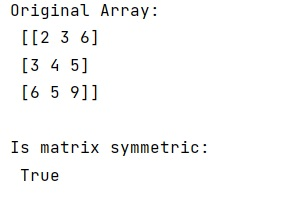# How to check if a matrix is symmetric in NumPy?

Given a NumPy matrix, we have to whether it is a symmetric matrix or not.
Submitted by Pranit Sharma, on February 26, 2023

NumPy is an abbreviated form of Numerical Python. It is used for different types of scientific operations in python. Numpy is a vast library in python which is used for almost every kind of scientific or mathematical operation. It is itself an array which is a collection of various methods and functions for processing the arrays.

## Checking if a matrix is symmetric

Suppose that we are given a matrix and we need to create a function with the arguments (arr, tol=1e-8) that returns a boolean value that tells the user whether the matrix is symmetric (the symmetric matrix is equal to its transpose).

To check if a matrix is symmetric or not, we will first define a function inside which we will simply compare to its transpose using allclose.

The allclose returns True if two arrays are element-wise equal within a tolerance. The tolerance values are positive, typically very small numbers. The relative difference (rtol * abs(b)) and the absolute difference atol are added together to compare against the absolute difference between arr1 and arr2.

Let us understand with the help of an example,

## Python code to check if a matrix is symmetric in NumPy

```# Import numpy
import numpy as np

# Creating a numpy array
arr = np.array([[2,3,6],[3,4,5],[6,5,9]])

# Display original array
print("Original Array:\n",arr,"\n")

# Defining a function
def fun(arr, tol=1e-8):
return np.all(np.abs(arr-arr.T) < tol)

# Checking whether matrix is symmetric or not
res = fun(arr)

# Display result
print("Is matrix symmetric:\n",res)
```

Output:Languages: » C » C++ » C++ STL » Java » Data Structure » C#.Net » Android » Kotlin » SQL
Web Technologies: » PHP » Python » JavaScript » CSS » Ajax » Node.js » Web programming/HTML
Solved programs: » C » C++ » DS » Java » C#
Aptitude que. & ans.: » C » C++ » Java » DBMS
Interview que. & ans.: » C » Embedded C » Java » SEO » HR
CS Subjects: » CS Basics » O.S. » Networks » DBMS » Embedded Systems » Cloud Computing
» Machine learning » CS Organizations » Linux » DOS
More: » Articles » Puzzles » News/Updates